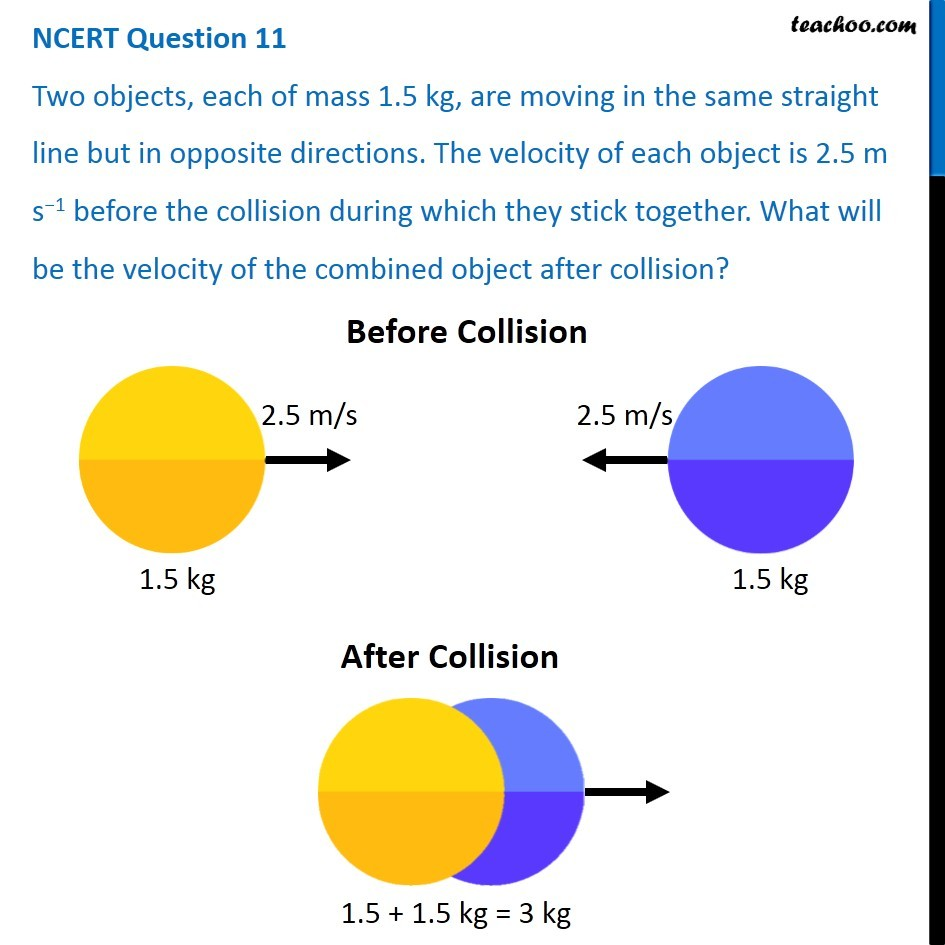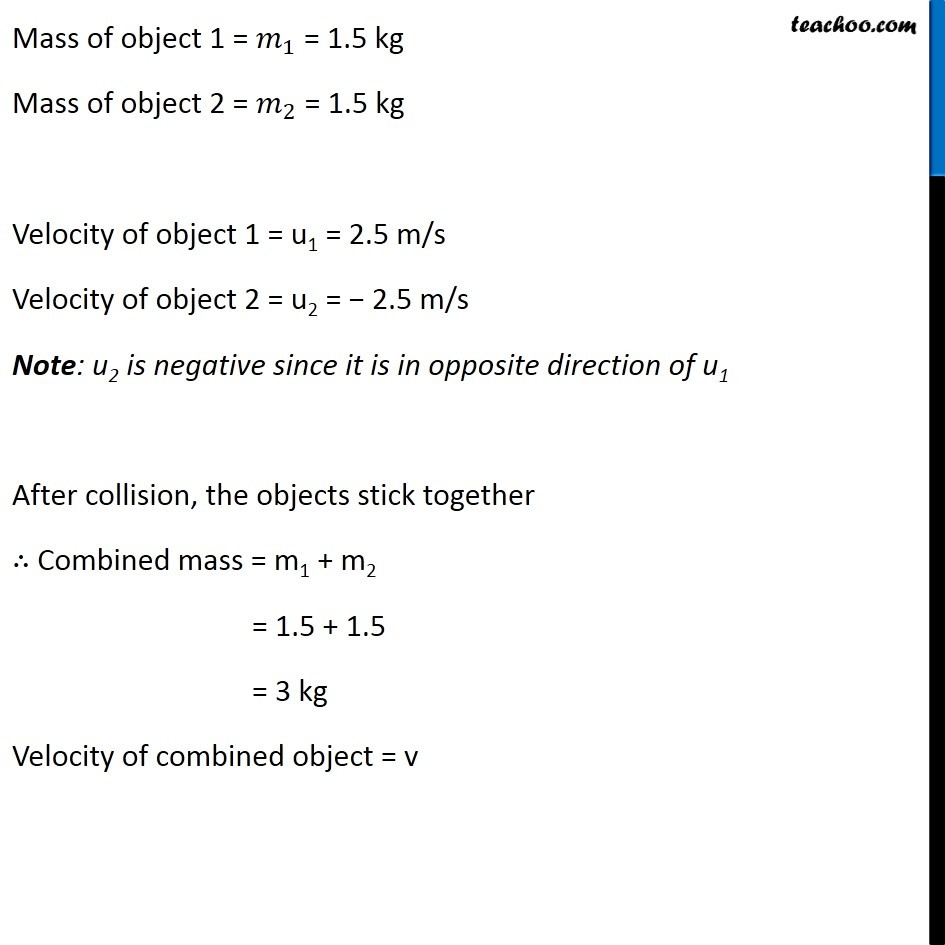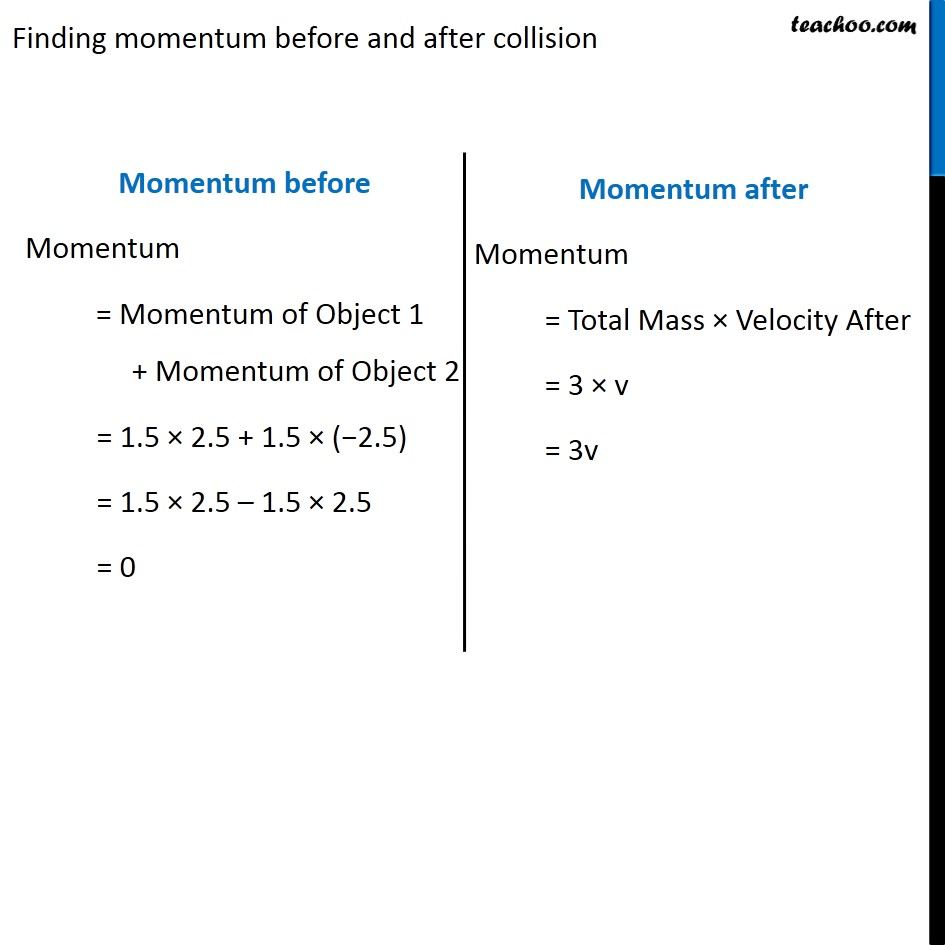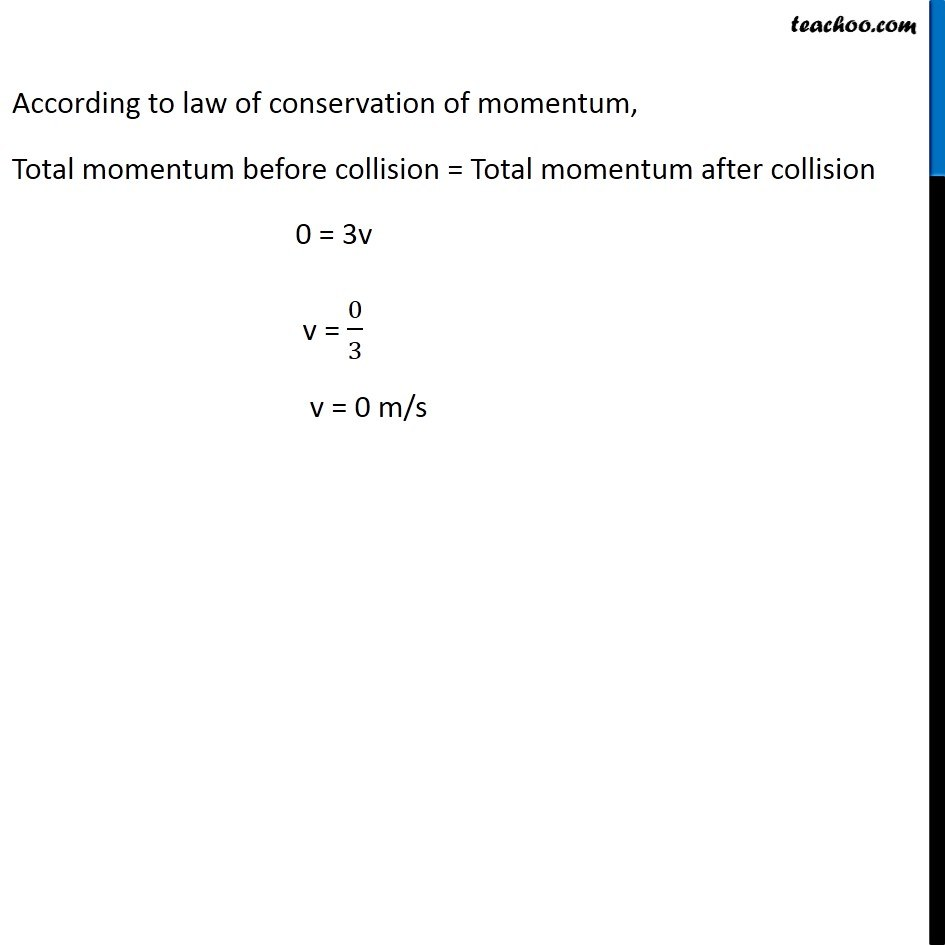NCERT Questions

Class 9
Chapter 9 Class 9 - Force and Laws Of MotionGet live Maths 1-on-1 Classs - Class 6 to 12

### Transcript

NCERT Question 11 Two objects, each of mass 1.5 kg, are moving in the same straight line but in opposite directions. The velocity of each object is 2.5 m s−1 before the collision during which they stick together. What will be the velocity of the combined object after collision? Mass of object 1 = 𝑚_1 = 1.5 kg Mass of object 2 = 𝑚_2 = 1.5 kg Velocity of object 1 = u1 = 2.5 m/s Velocity of object 2 = u2 = − 2.5 m/s Note: u2 is negative since it is in opposite direction of u1 After collision, the objects stick together ∴ Combined mass = m1 + m2 = 1.5 + 1.5 = 3 kg Velocity of combined object = v Finding momentum before and after collision Momentum before Momentum = Momentum of Object 1 + Momentum of Object 2 = 1.5 × 2.5 + 1.5 × (−2.5) = 1.5 × 2.5 – 1.5 × 2.5 = 0 Momentum after Momentum = Total Mass × Velocity After = 3 × v = 3v Momentum after Momentum = Total Mass × Velocity After = 3 × v = 3v According to law of conservation of momentum, Total momentum before collision = Total momentum after collision 0 = 3v v = 0/3 v = 0 m/s